Courses

# Time and Work - Examples (with Solutions), Arithmetic, Quantitative Aptitude Quant Notes | EduRev

## IBPS Clerk Prelims - Study Material, Mock Tests

Created by: Wizius Careers

## Banking Exams : Time and Work - Examples (with Solutions), Arithmetic, Quantitative Aptitude Quant Notes | EduRev

The document Time and Work - Examples (with Solutions), Arithmetic, Quantitative Aptitude Quant Notes | EduRev is a part of the Banking Exams Course IBPS Clerk Prelims - Study Material, Mock Tests.
All you need of Banking Exams at this link: Banking Exams

Introduction: Time And Work
Work is defined as something which has an effect or outcome; often the one desired or expected. The basic concept of Time and Work is similar to that across all Arithmetic topics, i.e. the concept of Proportionality.
Efficiency is inversely proportional to the Time taken when the amount of work done is constant.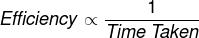This can be used to compare efficiencies and Time taken across different groups In Time-Speed-Distance, efficiency is replaced by Speed; i.e. Speed is inversely proportional to Time when the Distance is constant.Pipes and Cisterns are just an application of Time and Work. Concept wise, it is one and the same. In the above proportionality, Efficiency is replaced by Rate of filling. The equation in this case becomes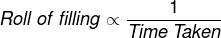The concept of percentage of work done can be used to solve most of the related questions.
A few basic points one needs to know to use the percentage concept are: When it is said that someone has done a work, it means he has done 100 % of the work. Hence, if A finishes a work in 4 days, it means- in 4 days he will do 100% of the work. Hence, in one day he finishes 25% (100/4) of the work. Similarly, in 3 days he finishes 75 % of the work.

TIME AND WORK SHORTCUT TRICKS
Table of commonly used numbers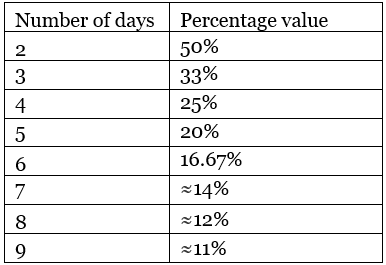The complete work can also be considered as 1 unit. Then if A takes 4 days to finish a work, it means he can finish 1/4th of the work in 1 day.

Illustrations: Here are some basic questions to illustrate time and work shortcut tricks.

Example 1. Rahim can finish a work in 10 days and Ram can finish the same work in 40 days. If Ram and Rahim both work together then what is the total number of days taken?
Solution.
The problem can be solved in two different approaches.
Approach 1: Using Fractions
Ram can finish the work in 10 days i.e. in one day he will do 1/10th of the work.
Rahim can finish the work in 40 days i.e. in one day he will do 1/40th of the work.
So, in one day, both working together can finish= (1/10) + (1/40) = 5/40 = 1/8th of the work. So, to complete the work they will take 8 days.
Approach 2: Using Percentage (Shortcut- Recommended)
Rahim can finish 100 % of work in 10 days i.e. in one day he finishes 10% of the work.
Ram can finish 100% of the work in 40 days i.e. in one day he finishes 2.5 % of the work.
So, working together, in a single day they can finish 12.5% of the work. So, to complete 100% of the work, they will take 100/12.5 = 8 days.

Example 2. Ravi can do a job in 10 days. Raman can do the same job in 20 days. They together start doing the job but after 4 days Raman leaves. How many more days will be required by Ravi to complete this job alone?
Solution.
Ravi can finish a job in 10 days i.e in one day he can finish 10 % of the job.
Raman can finish the same job in 20 days i.e. in one day he can finish 5 % of the job.
So, working together, in a day they can do 10 + 5 = 15 % of the job.
In the 4 days, if they worked together, they would have finished 4 × 15% = 60% of the job.
So, job left = 100 – 60 = 40%. This work has to be done by Ravi who does 10 % of the job in a day. So, to finish the remaining 40%, he will take 40/ 10 = 4 more days.

Introduction: Negative Work
Negative work increases the Time in which a work is to be completed. This application can be extended to cases involving Pipes and cisterns. Suppose there are two pipes in a Cistern. Pipe A is used to fill the Cistern and Pipe B is used to empty the Cistern. Here we say that Pipe B and Pipe A are working against each other. When a leak is developed in the Cistern, the leak forms the component of negative work, which slows down the completion of the task (in this case, the filling of the Cistern)
The following questions will explain the concept better.

ILLUSTRATIONS
Example 1. Pipe A can fill a tank in ‘an’ hours. On account of a leak at the bottom of the tank, it takes thrice as long to fill the tank. How long will the leak at the bottom of the tank take to empty a full tank, when pipe A is kept closed?
(a) (3/2)hours
(b) (2/3)hours
(c) (4/3)hours
(d) (3/4)hours
Solution.
Method 1: Using variables
The pipe can fill (1/a)th of the tank in an hour. Because of the leak, it can only fill 1/3a of the tank per hour. Let X be the time in which the leak can completely empty the tank, hence
1/x=1/a-1/3a = x =(3a/2)hrs.

Method 2: Using Numbers (Shortcut)
Assume a value for “a”- say 10 hours. Because of the leak, it will take 30 hours. Now, this means that in these 30 hours, the filling will occur at a rate of 3.33% and the leaking will slow down the process by 6.66% every hour Thus, the Time taken to empty a full tank= 100/6.66= 15 hours.
Answer: Only option (a) satisfies this value.

Example 2. A Cistern has three pipes A, B, and C. Pipe A can fill a Cistern in 10 hrs, Pipe B can fill a Cistern in 5 hrs while Pipe C can empty the Cistern in 20 hrs. If they are switched on at the same Time; in how many hours will the Cistern be filled?
Solution.
In one hour Pipe A can fill 100/10 = 10% of the Cistern.
In one hour Pipe B can fill 100/5 = 20 % of the Cistern.
In one hour Pipe C can empty 100/20 = 5 % of the Cistern.
If all three are working together, (10 + 20 – 5) = 25% of the Cistern will get filled in one hour, so it will take 4 hrs for the Cistern to fill.

Example 3. A tank can be filled by tap A in 6 hrs and by tap B in 3 hrs. But when they are open simultaneously to fill an empty tank they take 3 hrs more than their normal Time. A hole is later discovered as the reason for the delay. Find the Time taken by the hole to empty the tank if it is completely filled.
Solutions.
Tap A takes 6 hrs to fill, so in one hour it will fill 16.67 % of the tank. Tap B takes 3 hrs to fill, so in one hour it will fill 33.33% of the tank. Together they will fill 16.67% + 33.33% = 50 % of the tank. They should take 100/50= 2 hrs to fill the tank. But they take 3 hrs more, because of the hole; they totally take 5 hrs to fill i.e. they fill 20% in an hour.
This is possible if the hole empties 50-20 = 30 % of the tank in an hour. So to completely empty the full tank; the hole will take 100/30 hrs = 3.33 hrs = 3 hrs and 20 mins.

Inverse Proportionality of Efficiency and Time Taken
If the product of the two variables is always constant, the two are said to be inversely proportional.
Efficiency and Time taken are inversely proportional implies that If A is twice as good as B then A will take half the Time that B will take.
If the efficiencies are in the ratio m: n then Time taken will be in the ratio, n: m. i.e. If A is thrice is as good as B then
A will take (1/3)rd of the Time.

This can be proved with the help of the following proportionality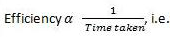If you are comparing the efficiencies and Time taken by two people,
EHA x TimeA = EHB x TimeB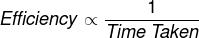ILLUSTRATIONS
Example 1. C takes 30 days to finish a work and A is thrice as good as C and twice as good as B. If A, B, and C work together, then how many days they will take to finish the work?
Solutions.
Approach 1: C takes 30 days to finish the work. Since A is thrice as good as C, A will take 10 days to finish the work Since A is twice as good as B, B will take 20 days to finish the work. Together A, B, and C will take 1/(1/10+1/20+1/30) = 5.4545 days.

Approach 2: Using Percentages (Shortcut)
1 day = 3.33% work by C
1 day= 10% work by A
1 day= 5% work by B
Together, in one day = 18.33% of work. Thus they will take 100/18.33 days= 5.4545

Example 2. A is twice as efficient as B. if they take 6 days to finish a certain job together, how much Time will they individually take to complete the work?
Solution.
Using the percentage approach It is given that A is twice as efficient as B. If they are finishing the work in 6 days, then in one day they will do 100/6 % of work= 16.66% as A is twice as efficient, work done by A in one day will be twice the work done by B. So work done by A in one day = 2/3 * 100/6 % = 100/9 % = 11.11% Work done by B in one day = 100/18 % = 5.55%. So, A will take 9 days to finish the job individually while B will take 18 days.

To start with, let’s take an example. If 2 men take 10 days to build a wall, 1 man will take 20 days (not 5 days) to build the same wall. The question arises Why?

The reason is simple that man and time are inversely proportional to each other. When men will decrease the number of days required to complete the work will increase. Here men become half, so time will double and will complete the work in 20 days.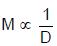Where M & D are number of men and number of days respectively.
If we further break number of days to hours, then total hours = DH, where H are number of hours per day.
Now, our formula becomes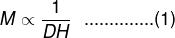To elaborate it further, let’s say M men take D days to build a room. Now if work is doubled (they have to build two rooms of same size) in same D no. of days, obviously they have to double their strength Or we can say that no. of men are directly proportional to the work to be finished. In mathematics, we can write it as
M ∝ W, where W is the work to be finished …………….. (2)
By combining (1) and (2), we get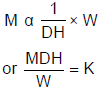Where K is constant of proportionality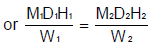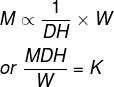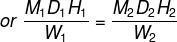This is our general formula to solve time & work problems. It is also known as Work Equivalence Method.
Majority of time & work questions can be divided into two types.

 TIP If a worker tokes x days to finish a work (working alone) and second one takes y days to finish the same work (working alone), then together they will take (xy/x+y) days to finish the same job.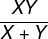Type I: Where efficiency of individual’s is not mentioned (as in the above example): These are the cases where the man-days concept is utilized. Here the Rule of Fractions or the proportion (direct or indirect) concept can also be used.
Type II: Where the efficiency is mentioned. Here the case of unit day’s or one day’s concept is utilized.

Concept of unit time
If A does a work in 10 days, then what is the one-day work done by A?
The answer is one-tenth part of the total work. We can say that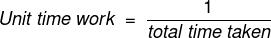Work done in unit time is known as efficiency of the worker or we can say, that if a worker takes less number of days (than second worker) to finish a work, he will be more efficient.

LCM Approach
In this type of approach the total work is considered as the LCM of the time taken by the individuals and then unit time work is calculated. Here the work done is not 1(unity) but the LCM of the time taken by number of persons.
Let us understand this with the help of an example.

Example 1. If Manni and Gopi finish a work in 10 and 15 days respectively, what will be number of days taken by both of them to complete the work when both work together?
Solution.
Let the total work is equal to the LCM of number of days of Manni & Gopi taken to do the work respectively.
Work = LCM of 10 & 15 = 30 units
One day work of Manni = 30/10 = 3 units
One day work of Gopi = 30/15 = 2 units
One day work of both = 5 units
So number of days taken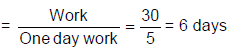Simultaneous Working Problems
It is always a good way if we try to solve such type of questions with the help of LCM approach. Let us understand the concept with the help of an example.

Example 2. A, B and C can finish a work independently in 10, 12 and 15 days. C starts the work and after 1 day, B joins him. After 1 day of B, A also joins them but leave 3 days before completion of the work, while B left two days before completion of work. What will be the total number of days taken to complete the work?
Solution.
Let total work is LCM (10, 12, 15) = 60 units
I day work: A = 60/10 = 6 units, B = 60/12 = 5 units, C = 60/15 = 4 units
Let total days taken = N
Days of A = (N - 5), Days of B = (N - 3), Days of C = N
According to the question we have,
6(N - 5) + 5(N - 3) + 4N = 60
Solving we have N = 7 days

Alternate Days concept
In these, type of problems, we see the work done by two or more men on alternate days or hours.

Example 3. A can build a wall in 20 days while B can build the same wall in 30 days. If they work on alternate days in how many days, will the wall be completed if A start the job?
Solution.
Let total work = 60 units
1 day work of A = 3 units and 1 day work of B = 2 units
2 days work = 3 + 2 = 5 units
To do 60 units, days required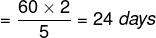Negative Work Concept
These types of problems revel about the problems of pipe and cistern in which one is inlet and other is outlet.
Let us solve a puzzle “A monkey wants to climb a pole which is 24 m tall. In 1 minute, he can climb up by 3 m and in the next minute, he slips by 2 m. In how many minutes will the monkey reach the top of the pole?

Example 4. If A build the wall in 20 days and B can destroy that wall in 30 days and work on alternate days. What will be the number of days required to build the wall for the first time?
Solution.
A can build 1/20 th of the wall in 1 day. Where as B will destroy 1/30 th of the wall in 1 day and since they are working on alternate days, So in 2 days, 1/20-1/30 = 1/60 th of the wall will be constructed.
So, in 57 × 2 = 114 days,  57/60th of the work will be completed and on the 115th day,
A will come and completes the remaining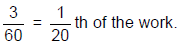So it takes 115 days to construct the wall.

Pipes and Cisterns
Pipes and Cisterns problems are almost the same as those of Time and Work problems. Thus, if a pipe fills a tank in 6 hrs, then the pipe fills 1/6th of the tank in 1 hour. The only difference with Pipes and Cisterns problems is that there are outlets as well as inlets. Thus, there are agents (the outlets) which perform negative work too. The rest of the process is almost similar.

Inlet: A pipe connected with a tank (or a cistern or a reservoir) is called an inlet, if it fills the tank. The work done by this is taken as positive work.

Outlet: A pipe connected with a tank is called an, outlet, if it empties the tank and the work done by this is taken as the negative work.

Example 5. Two pipes A and B can fill a tank in 36 hours and 45 hours respectively. If both the pipes are opened simultaneously, how much time will be taken to fill the tank?
Solution.
Part filled by A alone in 1 hour = 1/36
Part filled by B alone in 1 hour = 1/45
∴ Part filled by (A + B) in 1 hour =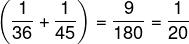Hence, both the pipes together will fill the tank in 20 hours.

Example 6. Pipe A can fill a tank in 36 hours and pipe B can empty it in 45 hours. If both the pipes are opened simultaneously, how much time will be taken to fill the tank?
Solution.
Total Volume of the tank = 180 units (LCM of 36 and 45)
1 hour work of A = 5 units
1 hour work of B = - 4 units
1 hour work of A + B = 1 units
So, Total time taken = 180 hours

Example 7. A can do a work in 30 days, and B can do in 40 days. If A and B work together for 10 days and A left, then C joined with B and completed the work in 10 days. How many days C alone can complete the work?
Solution.
A work only for 10 days, B work for 20 days and C work for 10 days.
Let us assume C require X days to complete the work.
So, in 1 day, A can do 1/30th of the work. B can do 1/40 th of the work, and c can do 1/X of the work.
So, 10/30 + 20/40 + 10/X = 1. So X = 60 days.
So, C can do the work in 60 days.

Example 8. Four men can do work in 15 days. If a man left the work after 5 days and again joined after 5 more days, The remaining three work continuously till the end of work. How many more days than the estimated, it takes to complete the work?
Solution.
After 15 days, the work that is left is equal to the amount of work, that can be done by 1 man in 5 days.
This work can be done in 5/4 days by the 4 men. So it takes 5/4 more days to complete the work.

Example 9. One man started a work on first day, the second day one more joined with him. The next day one more joined. Everyday one new person joined until the work gets completed. If the work is
completed in 15 days, how many days it takes for 10 men to complete the same work, if they work regularly?

Solution.
Let us assume one man can do x amount of work in 1 day.
So the amount of work, that can be completed
On 1st day = x
2nd day = 2x
……………..
..……………
15th day = 15x
Total = Σ15 x = 120 x
But 10 men can do 10x work in 1 day.
So, 10 men take 12 days to complete the work.

Example 10. A is 25% more efficient than B. With the help of C, B can complete a work in 2/3 times that required for A alone. How much percent less efficient is C, compared to A.
Solution.
Assume B can do ‘x’ work in 1 day.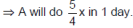Since B and C together take 2/3rd of the time required for A, (B + C) is 3/2 times effcient than A.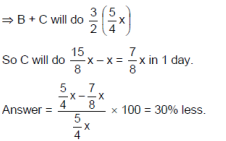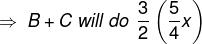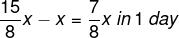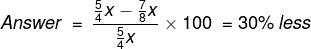Offer running on EduRev: Apply code STAYHOME200 to get INR 200 off on our premium plan EduRev Infinity!

## IBPS Clerk Prelims - Study Material, Mock Tests

74 videos|44 docs|81 tests

,

,

,

,

,

,

,

,

,

,

,

,

,

,

,

,

,

,

,

,

,

,

,

,

,

,

,

;